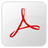## Math

*********

We will cover five areas of Mathematics: Number Sense, Algebra and Functions, Measurement and Geometry, Statistics, Data Analysis and Probability and Mathematical Reasoning.

Number Sense Standards

1.0 Students understand and use numbers up to 100:

1.1 Count, read, and write whole numbers to 100.

1.2 Compare and order whole numbers to 100 by using the symbols for less than, equal to, or greater  than (<, =, >).

1.3 Represent equivalent forms of the same number through the use of physical models,
diagrams, and number expressions (to 20) (e.g., 8 may be represented as 4 + 4, 5 + 3, 2 + 2 + 2 + 2,  10 − 2, 11 − 3).

1.4 Count and group object in ones and tens (e.g., three groups of 10 and 4 equals 34, or 30 + 4).

1.5 Identify and know the value of coins and show different combinations of coins that equal the same value.

2.0 Students demonstrate the meaning of addition and subtraction and use these operations to solve problems:

2.1 Know the addition facts (sums to 20) and the corresponding subtraction facts and commit them to memory.

2.2 Use the inverse relationship between addition and subtraction to solve problems.

2.3 Identify one more than, one less than, 10 more than, and 10 less than a given number.

2.4 Count by 2s, 5s, and 10s to 100.

2.5 Show the meaning of addition (putting together, increasing) and subtraction (taking away, comparing, finding the difference).

2.6 Solve addition and subtraction problems with one- and two-digit numbers (e.g., 5 + 58 = __).

2.7 Find the sum of three one-digit numbers.

3.0 Students use estimation strategies in computation and problem solving that involve numbers that use the ones, tens, and hundreds places:

3.1 Make reasonable estimates when comparing larger or smaller numbers.

**************************************************

Algebra and Functions Standards

1.0 Students use number sentences with operational symbols and expressions to solve problems:

1.1 Write and solve number sentences from problem situations that express relationships
involving addition and subtraction.

1.2 Understand the meaning of the symbols +, −, =.

1.3 Create problem situations that might lead to given number sentences involving addition and subtraction.

**************************************************

Measurement and Geometry Standards

1.0 Students use direct comparison and nonstandard units to describe the measurements
of objects:

1.1 Compare the length, weight, and volume of two or more objects by using direct comparison or a nonstandard unit.

1.2 Tell time to the nearest half hour and relate time to events (e.g., before/after, shorter/longer).

2.0 Students identify common geometric figures, classify them by common attributes, and describe their relative position or their location in space:

2.1 Identify, describe, and compare triangles, rectangles, squares, and circles, including the faces of three-dimensional objects.

2.2 Classify familiar plane and solid objects by common attributes, such as color, position, shape, size, roundness, or number of corners, and explain which attributes are being used for classification.

2.3 Give and follow directions about location.

2.4 Arrange and describe objects in space by proximity, position, and direction (e.g., near, far, below, above, up, down, behind, in front of, next to, left or right of).

**************************************************

Statistics, Data Analysis and Probability Standards

1.0 Students organize, represent, and compare data by category on simple graphs
and charts:

1.1Sort objects and data by common attributes and describe the categories.

1.2Represent and compare data (e.g., largest, smallest, most often, least often) by using
pictures, bar graphs, tally charts, and picture graphs.

2.0Students sort objects and create and describe patterns by numbers, shapes,
sizes, rhythms, or colors:

2.1 Describe, extend, and explain ways to get to a next element in simple repeating
patterns (e.g., rhythmic, numeric, color, and shape).

**************************************************

Mathematical Reasoning Standards

1.0 Students make decisions about how to set up a problem:

1.1 Determine the approach, materials, and strategies to be used.

1.2 Use tools, such as manipulatives or sketches, to model problems.

2.0 Students solve problems and justify their reasoning:

2.1 Explain the reasoning used and justify the procedures selected.

2.2 Make precise calculations and check the validity of the results from the context
of the problem.

3.0 Students note connections between one problem and another.

**************************************************

Flash Cards & Documentshundredschart1.pdf Size : 0.021 Kb Type : pdfaddition_subtraction_flashcards.pdf Size : 0.696 Kb Type : pdfalligators-poster.pdf Size : 0.12 Kb Type : pdfgreaterless-2digit.pdf Size : 0.045 Kb Type : pdftime-clock2.pdf Size : 0.167 Kb Type : pdfpennies.pdf Size : 0.039 Kb Type : pdfnickels.pdf Size : 0.036 Kb Type : pdfdimes.pdf Size : 0.04 Kb Type : pdfquarters.pdf Size : 0.034 Kb Type : pdf

This free website was made using Yola.

No HTML skills required. Build your website in minutes.

Go to www.yola.com and sign up today!

Make a free website with Yola